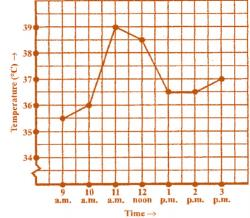Courses

Introduction To Graphs - MCQ

10 Questions MCQ Test Class 8 Mathematics by VP Classes | Introduction To Graphs - MCQ

Description
Attempt Introduction To Graphs - MCQ | 10 questions in 10 minutes | Mock test for Class 8 preparation | Free important questions MCQ to study Class 8 Mathematics by VP Classes for Class 8 Exam | Download free PDF with solutions
QUESTION: 1

Solution:
QUESTION: 2

Solution:
QUESTION: 3

The point (–5, 6) is nearer it:

Solution:
QUESTION: 4

The point (–3, –3) is

Solution:
QUESTION: 5

The point (0, 4) lies on which of the following:

Solution:
QUESTION: 6

The point (–3, 0) livs on which of the following?

Solution:
QUESTION: 7

The poits (–3, 2) and (2 , –3) represent:

Solution:
QUESTION: 8

By joining (– 1, –1) , (0, 0) and (3 , 3) which of the following is obatained?

Solution:
QUESTION: 9

By joining (–3 , 2) , (–3 , –3) and (–3, 4), which of the following is obtained?

Solution:
QUESTION: 10

Which of the following points lies on y-axis?

Solution:Use Code STAYHOME200 and get INR 200 additional OFF Use Coupon Code

Track your progress, build streaks, highlight & save important lessons and more!

Similar ContentRelated tests